# Misprint experiments

This page describes experiments and presents results for the HP Misprint Detection project.

#  Theoretical method

Two results are interesting with the theoretical experiments:

The first results are we the noise model described in the theoretical paragraph: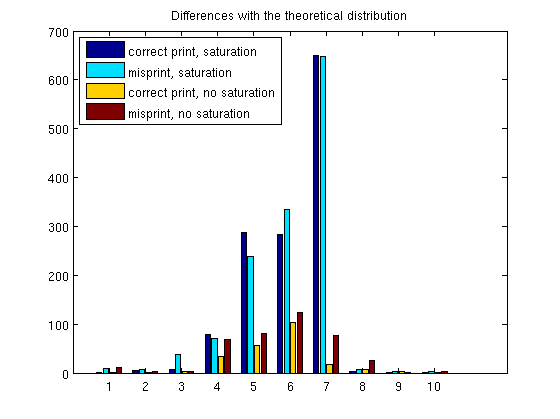Theoretical approach, histogram of the differences with the theoretical distribution

On this graph only the correct print without saturation would is accepted with a p-value of 32%. All the other images are rejected, with a p-value of 0 (with MATLAB finite precision).

The method would be extremely effective with a perfect simulation, and that the model implemented by the simulation is actually correct. Here we could have a correct model, but it depends on the image, as it depends on which values are saturated. Computing the theoretical histogram would require to compute a weighted histogram of the different deviation distribution. Even though it would be possible, and it would probably solve the problem for the simulations, it would not sole the high level problem: our simulator is not likely to be a extremely precise simulator of the reality. We have to deal with that fact.

#  Learning method

These results are for the general method previously described in the Misprint page. Once the conditional distributions of probability are learned, we can use them to infer the posterior probability of having a misprint given all the statistics that we computed on a given image.

##  Conditional Distributions of Probability

We can first take a look at these distributions for the statistics as they give us an idea of how, and how well, the algorithm will be working:

You will find here 2 sets of curves: the first set comes from our early experiment, the second set comes from the same algorithm, but with some tuned parameters.

##  First CDP Tables

These probabilities were computed with our largest training set so far of 999 images. The quantization step is also adaptated to the range of the actual values, so ths x-axis just means i-th quantization value.

Here, the curves represent P(correct print | stat) We can see that these 2 statistics are working quite well, as they are quite discriminant between misprint and correct print: most of the values are close to 0 and 1. We can also check that the value make sens as:

• For the fraction statistic, we have a low probability of having a high proportion of the values lower that 0.5
• For the mean value, the high probability of correct print is when the mean is high.

We can also look directly at the different CPDs: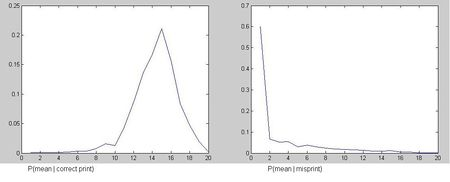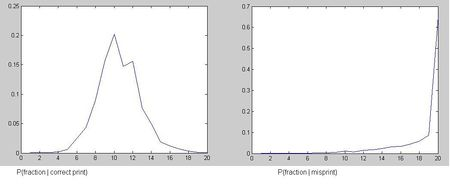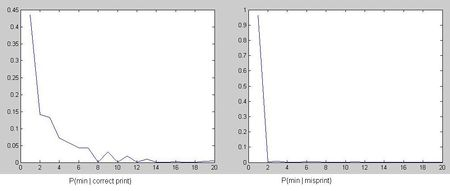CDP Table for the mean feature CDP Table for the fractional feature CDP Table for the minimum feature

We can see on these curves that the CPDs of the mean and the fraction gives us a nice differenciation. but the the quantization table of the CPD of the min statistic would need to be reshaped to be more useful.

##  Second set of tables

After some changes in the algorithm: better look up tables, changing the quantization of the features and fixing some bugs, we can take a new look at the conditional distribution of probability for each feature.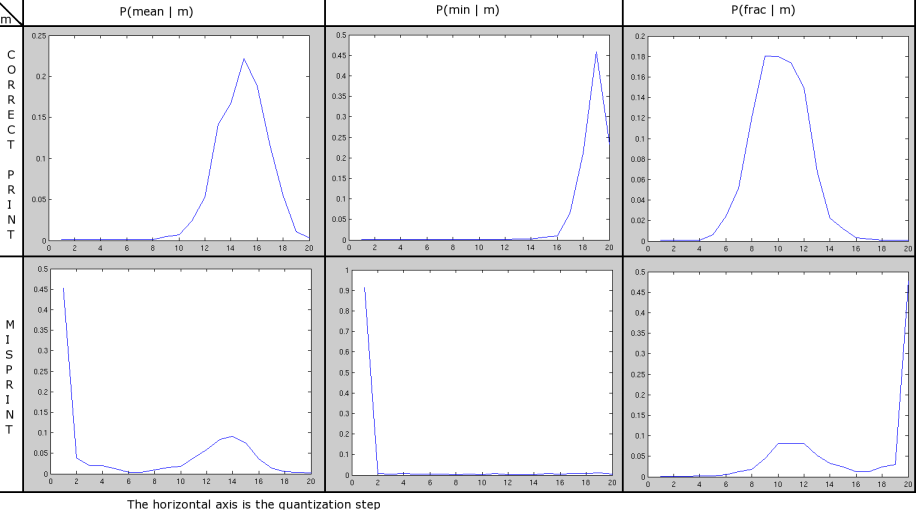Secoind set of CDP Tables

We can see know that the 3 features give a nice separation between the correct print and the misprinted case: in each case the high values, i.e. the peak, are on completely different quantization steps, which means that when the value of the feature is computed it is very likely to induce a high probability for one case and a low probability for the other case. This is what we want to be able to distinguish between the correct case and the misprinted case.

• The min table is the one with the strongest separation: two cases are highly dominating: the first step for the misprinted case as one of the window was absolutely not what it should be. and the last step for the correct case: if the page is correct, the window with the smallest probability stays high enough.
• The mean and the frac table are very similar: in the normal case, both have a nice peak around the 15th step for the mean and the 10th step for the frac feature, and the peak for the misprinted case is on an extreme step, far from the normal values. But for these two features, there is also a small but non negligible rebound very close to where the normal peak is. This lowers the efficiency of this feature in general. It can be explain by a simple remark: we have basically 2 types of misprints: local misprints and widespread misprints. In the case of a local misprint, the differences between the two output (simulated and actual) barely affects the mean and the frac features as these reflect the general behaviour of the features over all the windows. This cases create this rebound.

To solve the problem mention in the second point, a solution could be to have to different tables, one calculating the probability of having a small localized misprint and the other for widespread misprints.

#  Algorithm performance

Then we can use these tables on test data, to see how well they perform.

Here you can see the evolution of our results, from the early simulations to the latest experiments. Some changes were made in between, like better look up tables, some bug fixing, tuned parameters...

##  First set of results

These experiments were run with only one type of misprint: the missing plane, but the plane choice was random.

We trained and tested with 2 data sets of different sizes, and on these results, we can easily see the improvement that we have with a bigger data set.

First training set : 109 images, first testing set: 33 images, this gives us these ROC curves / Precision-Recall curves: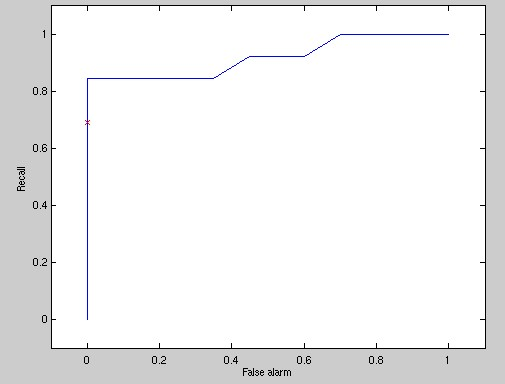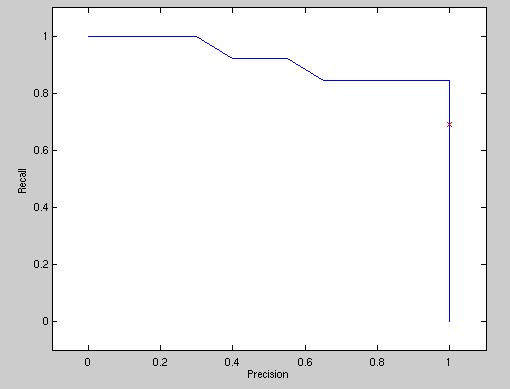First ROC Curve First Precision-Recall Curve

These results are quite promising given the small training set. We can now train and test the system on 10 times more images.

The curves below show some results that are much better and probably closer to the capacities of the system, as the training was done on 999 images, and the testing on 339 images. Some improvement probably also comes from a change in the quantization of the statistics: while previously we were using a uniform quantization on [0,1], it is now adaptated to the range of the actual values taken by the statistics (for instance, the minimum higher than 0.2 almost never happen...).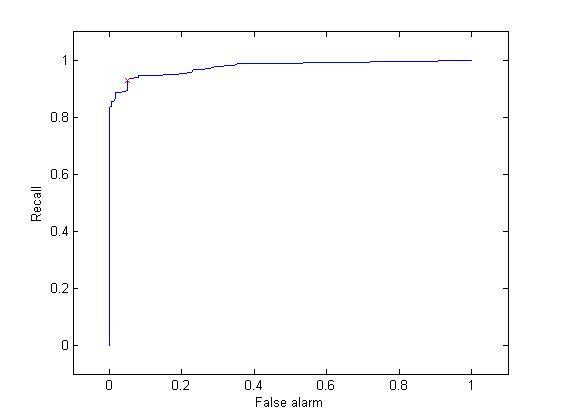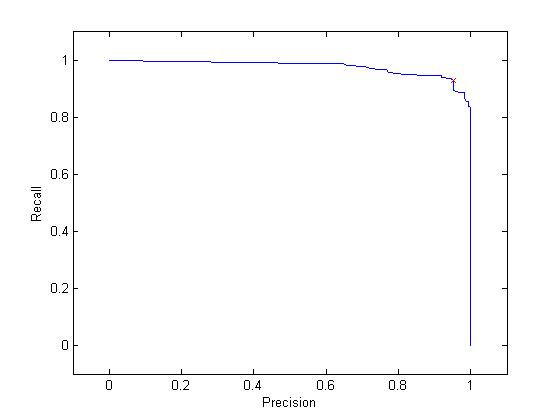ROC Curve with the whole set Precision-Recall Curve with the whole set

We can see that these results are much better, and almost perfect, as we are very close to the 1 line for the whole curve, and the turn to 0 is abrupt. Of course, this is only for one type of misprint: the missing colour plan, but the sensor is monochromatic, and the noise settings are quite high (much higher that what they would be in reality), but one can think that it might compensate for some inaccuracies of the model.

##  Results after some modifications

Results for a better look up table and a better conversion to cmyk - results for different misprint types: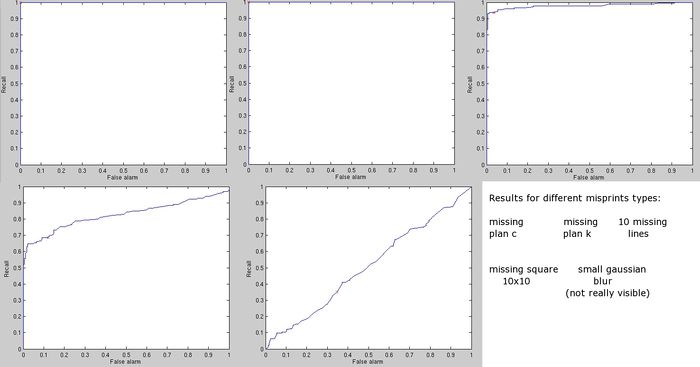ROC Curves: Comparison between different misprints

We can see now that for the wide spread errors, misprints were always caught on the 300 test images and all the good prints passed the tests, the ROC curves for these two misprints are indeed perfect. The results for the missing lines are also very good: they are almost perfect, only a few errors were made.

The results for the 2 other misprint types are not that good, but they are at the edge of the system possibilities: the missing square is a 10x10 pixels square missing in the middle of the image, this only represents at most a 2x2 error on the sensor image. To catch these problems, a combination of different parameters for the sliding window, and a less noisy sensor are probably needed... simulations are running. A standard image size could also be helpful as it would give more consistent data to the learning algorithm.

The last results with the blurring are really bad. But the blurring parameters are probably not high enough as I could not see the difference between the correctly printed page and the misprinted page on the example that I tried.

##  Effect of the noise

The sensor on which we based our experiments is highly noisy, this clearly creates more difficulty to distinguish between a misprint and a correct print as it gives a wider range of likely values for each CMYK, and therefore creates more overlap between different values.

As the wide spread errors are easily found by the algorithm (you can see on the results part), even when the noise is quite high, it is interesting to see the effect of the noise on the localized misprints, like the missing square:

The following ROC curves show this effect of the noise, as expected, with a lower noise, the algorithm performs very well on the missing square. Even while the features are computed bbby averaging on big windows.

The variation of the accuracy of the method varies with the noise as we would expect: less noise implies better results.

##  Effect of the window size

One other experiment is to vary the window size (see this page for more details about this parameter). This is the only magical parameter that is in the whole algorithm. A bigger window averages more the effect of the noise, and therefore should be more efficient at detecting small widespread defects, particularly for a noisy sensor. A smaller window should be more sensible to the noise, but should be more efficient in spotting small localized misprints.

In the following experiment, we varied the widow size (for a squared window from 5x5 pixels to 30x30 pixels), with a fixed noise of 0.6, the misprint tested is a small missing square in the image, which is a misprint poorly catch with the big windows as the previous experiments have shown.

We can see that the results behave as expected: with a smaller window, the algorithm becomes more and more efficient in catching the misprints: the 5x5 window with 60% or the noise is even much more efficient than the original (much biggest) window, with a 20% noise. One can also remark that for the 0.5 threshold (the red cross), there are no false alarm. It is not clear if it is indeed a good point though we can think that it is better to leave the page with a very small misprint from time to time, rather than stopping the printing process for no reason too often.

#  Comparison of noise and parameters for the full model

The previous measurements come from computations with the first statistical model and with one sensor. While the following experiments come from simulations with 2 sensors and the second model separating global and local misprints.

There is one image of results for each noise level, mostly results are getting better when the noise is lower. It is however difficult to generalize small differences in these curves as there is a random factor in generating these results, and some curves are so close to each other that some small differences in the random outcomes could switch the order of the curves.

For this experiments, the tables were learned with 1042 images, and the tests performed on 294 images. You can find the table in cdpTables/ and the results in results/, the naming convention is: objectNoise_windowWidth_window_height.mat, for instance results08_5_5.mat means that these results were computed with a noise at 0.8 = 80%, and a window size of 5x5.

The ROC curves are computed choosing randomly for each image if there is a misprint or not. And if it does the misprint type is also chosen randomly.

You will find the details of each curves in the sections below, but we can sum up the results in the following graph:

</td> </td>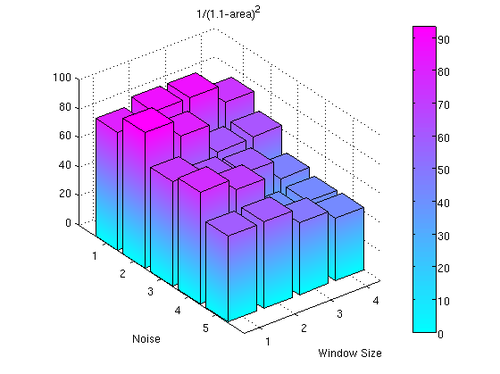Bars representing the area under the ROC Curves

To have a more readable graph, it is not directly the area under the curve that is plotted, but an increasing function of this area. Indeed, all these results being very close to 1, plotting directly the areas would make the plot unreadable.

There seems to be a downhill diagonal from (0,0) to (4,5), but because of some randomness in the results, it is difficult to find a net tendency. We are rerunning these tests on a larger database.

##  Noise at 20%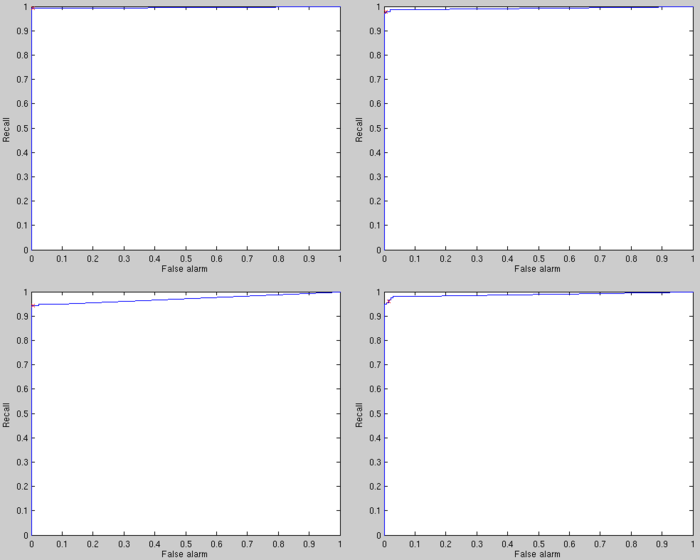Results for the noise at 20% and window size of kxk k=5 k=10 k=20 k=30

In the 5x5 case, there were two errors: one uncaught misprint, but by looking at the image, you cannot tell either that there is a misprint, and one false alarm

##  Noise at 40%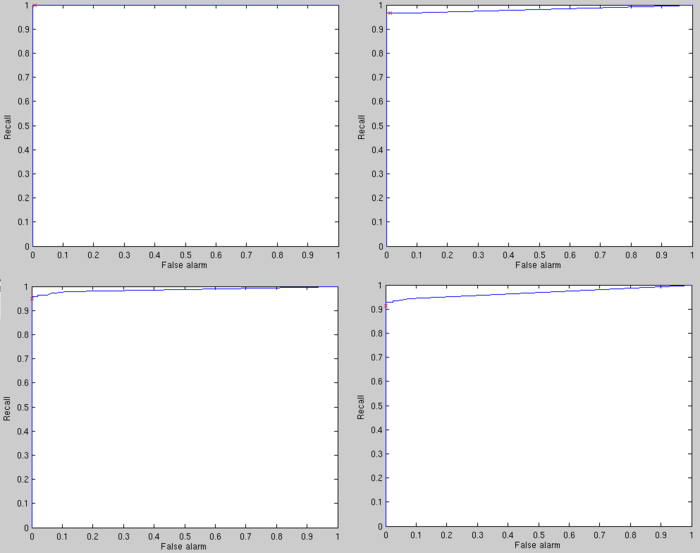Results for the noise at 40% and window size of kxk k=5 k=10 k=20 k=30

In the 5x5 case, ther were only 1 error with the threshold at 0.5, it was a false detection.

For the low noise values: 40% and 20% the results are almost perfect in both cases when using smaller windows (like the 5x5 window). These noise level are probably not completely realistic, but they give a good reference in term of accuracy: in spite of the very different misprint types that we have now, perfect results are almost reachable.

##  Noise at 60%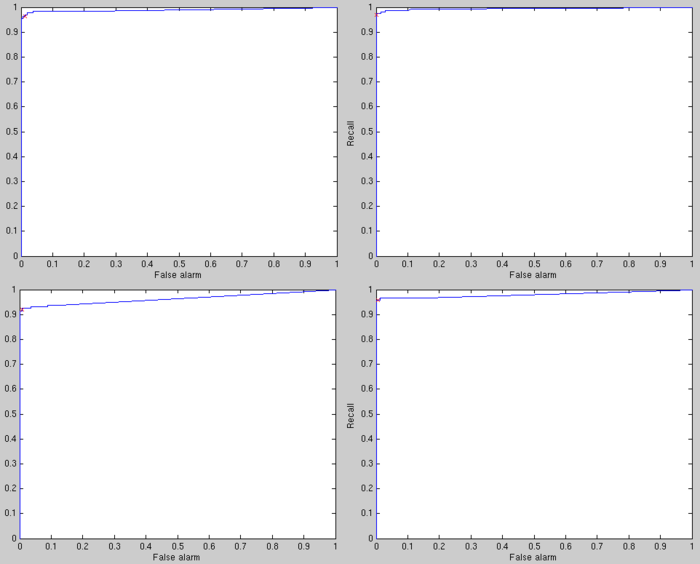Results for the noise at 60% and window size of kxk k=5 k=10 k=20 k=30

The best window size for such a noise level seems to be k between 5 and 10. It is quite surprising that k=30 gives better results than k=20.

The uncaught errors seem pretty difficult to detect, the following is one of these (arrived for k=5)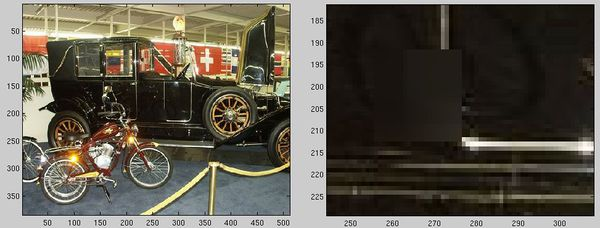Uncaught error example Whole image Zoom on the misprint

##  Noise at 80%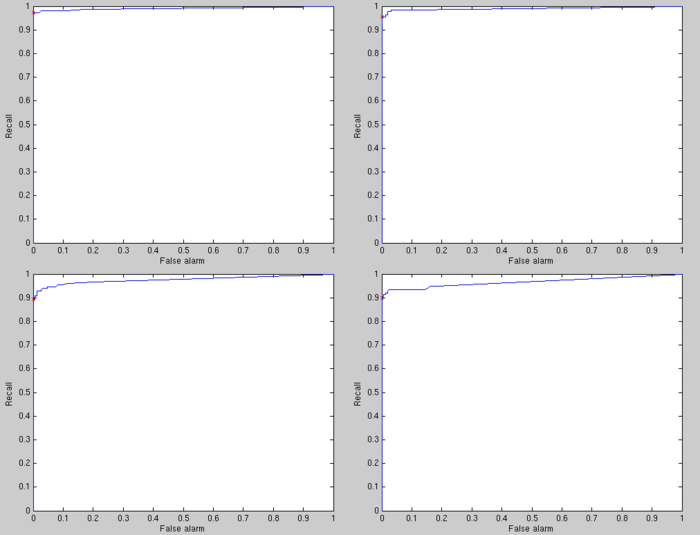Results for the noise at 80% and window size of kxk k=5 k=10 k=20 k=30

These results show that with these modifications, the algorithm becomes very efficient and makes only a few mistakes. Even with a quite high noise, the best results are smal a rather small window: k between 5 and 10 seems good.

##  Noise at 100%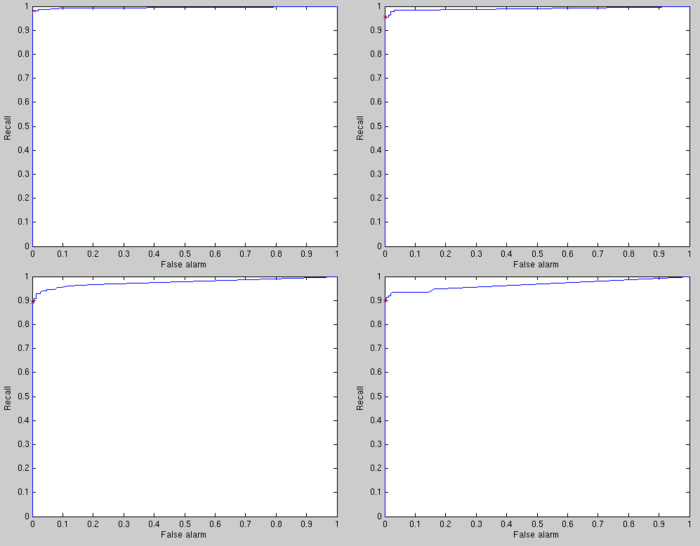Results for the noise at 100% and window size of kxk k=5 k=10 k=20 k=30

Even with a noise at 100% the results are still very good, the 2 changes made to the algorithm (different tables for global and local misprints and using 2 sensors) seem to be really efficient.

These results show that the system is working very well, and that adding another sensor can compensate very well for the noise, as even with these two pretty noisy sensors, we still have good results with rather small windows.

• The red cross is the point reached with a threshold of 0.5 to decide between misprint and correct print, the fact that this cross is very close to the upper left corner means that the 0.5 value is a pretty good threshold, and that the assumptions done previously in the model are probably not too false.
• If we want a system that has very few false alarm, and without missing important misprints, ther could be a neutral zone in which we are not deciding whether it is a misprint or not. For instance if the probability of a misprint is between 0.4 and 0.6, we do not decide (but we may send the picture somewhere for instance). That way, only small misprints would be missed and the false alarm rate could fall very low.

#  Comparison of the different systems

We can compare the different ROC curves obtain with our different settings of the algorithm (one or two sensors and the two statistical models). These results are all computed for a 100% noise:

At a first look, we can see that the 2 sensors, with the local/global misprint model gives the best performance. However, it is interesting to give a closer look at those curves, as they give a better understanding of how the interactions between the different parameters and the algorithm.

We can first take a closer look at the upper left curves: these are obtained for a window size of 5x5. We can see that the algorithm running with 2 sensors and the statistical model taking into account local and global misprints (red curve) outperforms every other combination. However it is closely followed by the black curve (algorithm running the 2 sensor with the simpler model), while the two other curves (blue and green), representing the system with only 1 sensor are far behind. This shows that much of the improvement comes from the second sensor and the increment of performance coming from the second model id quite small in comparison. This has yet to be compared with their computational cost: the complexity of the algorithm being linear in the number of sensor while switching between the two models costs only a few operations - completely negligible compared to the overall cost -. In other words, to go from blue-green to red-black, we need to double the number of operations, while it costs virtually nothing to go from green-black to blue-red. Therefore the improvement coming from the change of statistical model is not to be neglected as it is free.

By looking at the second curve (with a window size of 10x10), we can see that by widening the window, this reduces the difference between the 2 models. Indeed by averaging over a bigger window, it it removes the particularities of the local misprints that we are actually trying to catch. This is what one can expect as with too bog a window, the local misprints do not make any quantitative differences in the learned CDP: these tables then look like the correct print table.

There does not seem to be a general trend in the effect of the window size. It seems that having a bigger window leads to similar results between the different settings. These interactions between settings and window size are complicated as shows the last curve (window size of 10x10) on which the green curve is very different than the other curves.

#  Robustness of the learning to simulation error

To test the robustness of the learning to simulation error, we use two different simulators: one simulator represents reality, and the other represents the simulation. The difference introduced is that the simulator simulating the simulation does not implement the voltage swing. We saw previously that this introduces a significant distortion.

There are therefore 2 inaccuracies in the system: the simulator is not right, and the assumption of Gaussian noise in the normalization is still not correct.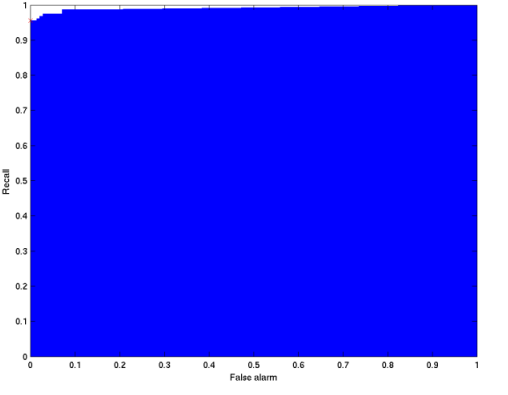Results with an imperfect simulator
We can see on this ROC curve that the learning algorithm still performs very with a imperfect simulator. We could hope to get even better results by tweaking the different parameters of the algorithm; particularly the feature quantization. This show that the learning method is a successful approach for this problem as we can not assume that the simulator is going to be perfect. This example shows that it seems reasonable to consider that we can have a working algorithm based on simulating the sensor output. Of course, the better the simulator, the better the results, so perfecting the simulation through real experiments would be a crucial step an industrial system.

#  Efficiency_vs_Computation

We can make an approximated evaluation of the computational power needed for this algorithm in a real time application by counting the number of operations needed in each step:

Considering that we have a MxM image, S sensors that are N pixels long, that the look-up tables sizes are (T1,T2,T3,T4) for the CMYK to output table and Q for the quantization table, then we need:

[itex]4SM^{2}+SN^{2}[5+log_{2}(T1)+log_{2}(T2)+log_{2}(T3)+log_{2}(T4)+3*log_{2}(Q)][/itex]

computations by pages, most of this time is spend in lookup tables. The time spend for the posterior inference calculation is completely negligible.

For a 2000x2000pixels image, and a 1000 pixel long sensor, with our current look-up tables and 2 sensors, this makes roughly 98 000 000 operations per page, which at a 10 pages per second rate is roughly 1,000,000,000 per second.

Therefore, we can consider that the order of magnitude of the computational power needed for this algorithm is 1GFlops. Moreover most of the costly computations here are highly parallelizable. This of course has to be understand as an order of magnitude to understand the hardware needed. This is to compare with today's GPU (running at about a 1000GFLOPS), CPU (running dozen GFLOPS per core -see Intel's page-).

The main factor here comes from Q, so one easy way to speed the computation would be to reshape the feature quantization tables with less quantization steps. Suppressing the fractional feature that is very close to the mean feature could also be a good idea. This would probably not affect to much the results.

#  Effect of misregistration

With the algorithm exactly as it is right now, the results are quite sensible to misregistration. This is not surprising as the pixel values of these two images are often different (they are surely much more different than some misprints that we want to detect):

</td> </td>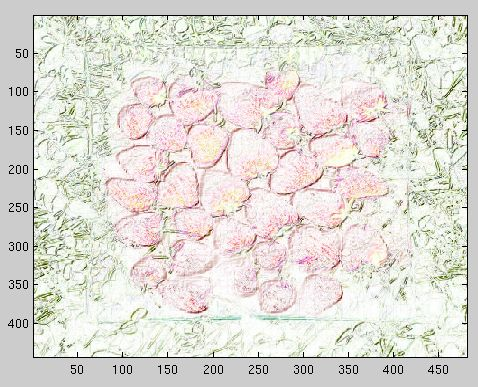Difference between original and misregistered image
We can first think that misregistration should not really be a real problem, as the sensor can be just after the printer's heads and so we should know exactly where is the image (not necessary on the page, but relatively to the sensor), and if the image drifted, it is probably a side that something is not working as it should. If it appears that misregistration is a problem, then first some fast registration algorithm could be tried. Then if this does not completely solve the problem, then some averaging is needed inside the algorithm (before the window step). Of course, this would probably have a great impact on the possibility of detecting small localized misprints.